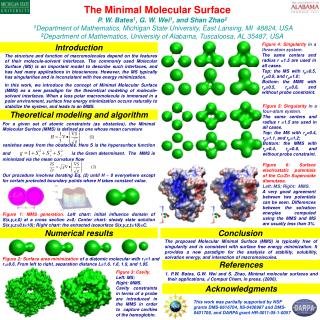DownloadDownload PresentationFigure 5: Singularity in a four-atom system. The same centers and radius r =1.5 are used in all cases.

# Figure 5: Singularity in a four-atom system. The same centers and radius r =1.5 are used in all cases.

Download Presentation## Figure 5: Singularity in a four-atom system. The same centers and radius r =1.5 are used in all cases.

- - - - - - - - - - - - - - - - - - - - - - - - - - - E N D - - - - - - - - - - - - - - - - - - - - - - - - - - -
##### Presentation Transcript

1. For a given set of atomic constraints (as obstacles), the Minimal Molecular Surface (MMS) is defined as one whose mean curvature vanishes away from the obstacles. Here S is the hypersurface function and is the Gram determinant.The MMS is minimized via the mean curvature flow Our procedure involves iterating Eq. (2) until H ~ 0 everywhere except for certain protected boundary points where H takes constant value. Figure 1: MMS generation. Left chart: initial influence domain of S(x,y,z,0) at a cross section z=0; Center chart: steady state solution S(x,y,z=0,t=10); Right chart: the extracted isosurface S(x,y,z,t=10)=C. The Minimal Molecular Surface P. W. Bates1, G. W. Wei1, and Shan Zhao21Department of Mathematics, Michigan State University, East Lansing, MI 48824, USA2Department of Mathematics, University of Alabama, Tuscaloosa, AL 35487, USA Figure 4: Singularity in a three-atom system. The same centers and radius r =1.5 are used in all cases. Top: the MS with rp=0.5, rp=0.9, and rp=1.0; Bottom: the MMS with rp=0.5, rp=0.6, and without probe constraint. Introduction The structure and function of macromolecules depend on the features of their molecule-solvent interfaces. The commonly used Molecular Surface (MS) is an important model to describe such interfaces, and has had many applications in biosciences. However, the MS typically has singularities and is inconsistent with free energy minimization. In this work, we introduce the concept of Minimal Molecular Surface (MMS) as a new paradigm for the theoretical modeling of molecule-solvent interfaces. When a less polar macromolecule is immersed in a polar environment, surface free energy minimization occurs naturally to stabilize the system, and leads to an MMS. Figure 5: Singularity in a four-atom system. The same centers and radius r =1.5 are used in all cases. Top: the MS with rp=0.4, rp=1.1, and rp=1.2; Bottom: the MMS with rp=0.4, rp=0.8, and without probe constraint. Theoretical modeling and algorithm Figure 6: Surface electrostatic potentials of the Cu/Zn Superoxide dismutase. Left: MS; Right: MMS. A very good agreement between two potentials can be seen. Differences between the solvation energies computed using the MMS and MS are usually less than 3%. Numerical results Conclusion The proposed Molecular Minimal Surface (MMS) is typically free of singularity and is consistent with surface free energy minimization. It provides a new paradigm for the analysis of stability, solubility, solvation energy, and interaction of macromolecules. Figure 2: Surface area minimization of a diatomic molecular with r1=1 and r2=0.6. From left to right, separation distance L=1.6, 1.8, 1.9, and 1.95. References Figure 3: Cavity. Left: MS; Right: MMS. Cavity constraints in terms of a probe are introduced in the MMS in order to capture cavities of the hemoglobin. 1. P.W. Bates, G.W. Wei and S. Zhao, Minimal molecular surfaces and their applications, J Comput Chem, in press, (2006). Acknowledgments This work was partially supported by NSF grants DMS-0616704, IIS-0430987 and DMS-0401708, and DARPA grant HR-0011-05-1-0057